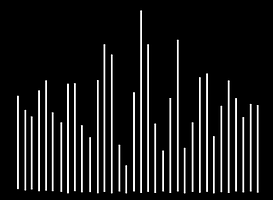top of page

## Converting Analogue Sound to Binary

Analogue sound waves must be digitally recorded and stored in binary.

To record the sound, the amplitude (height) of the analogue sound wave is measured and recorded in binary at specific intervals.

0010 1011 0101 0101

Analog sound wave

(Analog to Digital Converter)

Binary sample

## Sampling an Analogue Sound Wave

Digital sampling is discrete (separate) and not continuous like analogue waves.

To get the highest quality sound, many samples are taken to recreate the analogue wave as closely as possible.

## Sample Rate

The sample rate (sampling frequency) is the number of times per second the amplitude of the sound wave is measured. It is measured in kilohertz (kHz), for example CD quality is 44.1kHz (44,100 samples per second).

The higher the sample rate, the better the audio quality as the digital data more closely resembles an analogue wave.

However, higher sample rates result in larger file sizes because more data is stored for each individual sample.A low sample rate will result in a low-quality sound because the digital data does not closely resemble the original analog wave.A higher sample rate will result in a higher-quality sound because the digital data more closely resembles the original analog wave.

## Bit Depth

The bit depth is the number of bits available to represent each sample. For example, a sample with a bit depth of 4 could be 0101 or 0111 or 1010.  A sample with a bit depth of 8 could be  01010110 or 1010110 or 11001111. A common bit depth is 16 bits.

The higher the bit depth, the more bits are available to be used for each sample. Therefore the quality is often higher as the wave more closely resembles an analog wave.

The file size will also be larger if the bit depth is higher, as each sample stores additional bitslow bit rate = lower qualityhigh bit rate = higher quality

sound file size = sample rate x bit depth x duration

Example: A short audio sample has a bit depth of 4 and a sample rate of 10 samples per second. The clip is 15 seconds long.

4  bits x 10 = 40 bits per second. 40 x 15 = 600 bits.

To convert the answer from bits to bytes, divide by 8.

600 bits ÷ 8 = 75 bytes.

## Calculating File Size## Questo's Questions

2.4d - Sound Storage:

1. Explain how an analogue sound wave is converted into a binary sample

2a. What is a sample rate

2b. Explain two ways an audio file will be affected if the sample rate is increased

3a. What is bit depth

3b. Explain two ways an audio file will be affected if the bit depth is increased

4. An audio sample has a bit depth of 8, a sample rate of 10 and it is 12 seconds long. What is the file size in bytes

bottom of page